• This is an assessment test.
• These tests focus on geometry and mensuration and are meant to indicate your preparation level for the subject.
• Kindly take the tests in this series with a pre-defined schedule.

## Geometry and Mensuration: Test 22

Congratulations - you have completed Geometry and Mensuration: Test 22. You scored %%SCORE%% out of %%TOTAL%%. You correct answer percentage: %%PERCENTAGE%% . Your performance has been rated as %%RATING%%
 Question 1
AB= 8 cm and CD= 6 cm are two parallel chords on the same side of the centre of a circle. The distance between them is 1 cm. The radius of the circle is
 A 5 cm B 4 cm C 3 cm D 2 cm
Question 1 Explanation:$\begin{array}{l}Let\text{ }the\text{ }radius\text{ }be\text{ }x\text{ }cm.\\\sqrt{{{x}^{2}}-9}-\sqrt{{{x}^{2}}-16}=1\\Putting\,x=5,\\L.H.S=1=R.H.S\end{array}$
 Question 2
Two parallel chords are drawn in a circle of diameter 30 cm. The length of one chord is 24 cm and the distance between the two chords is 21 cm. The length of the other chord is
 A 10 cm B 18 cm C 12 cm D 16 cm
Question 2 Explanation: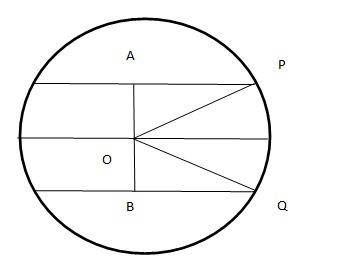Question 3
Chords AB and CD of a circle intersect externally at P. If AB= 6 cm, CD= 3 cm and PD= 5 cm, then the length of PB is
 A 5 cm B 7.35 cm C 6 cm D 4 cm
Question 3 Explanation: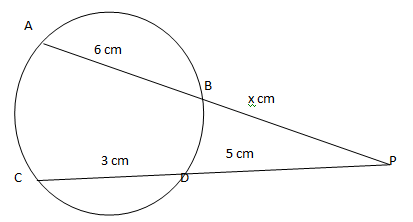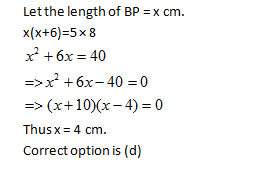Question 4
AB and CD are two parallel chords on the opposite sides of the circle $\displaystyle \overline{AB}=\text{ }10\text{ }cm,\,\,\overline{CD}=\text{ }24\text{ }cm$and the radius of the circle is 13cm, the distance between the chords is
 A 17 cm B 15 cm C 16 cm D 18 cm
Question 4 Explanation:$\begin{array}{l}Let\text{ }O\text{ }be\text{ }the\text{ }center\text{ }of\text{ }the\text{ }circle.\\OB=OD=13\text{ }cm\\Thus\text{ }the\text{ }distance\text{ }between\text{ }them\text{ }is\\\sqrt{{{13}^{2}}-{{5}^{2}}}+\sqrt{{{13}^{2}}-{{12}^{2}}}\\=12+5\\=17\end{array}$
 Question 5
The length of the common chord of two circles of radii 15 cm and 20 cm whose centres are 25 cm apart, is (in cm)
 A 12 B 24 C 36 D 48
Question 5 Explanation: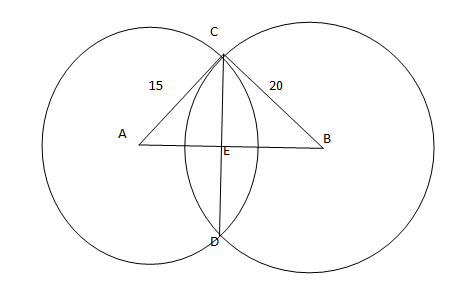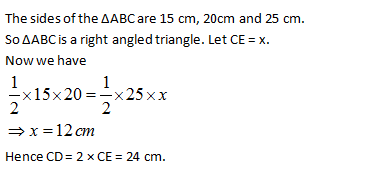Once you are finished, click the button below. Any items you have not completed will be marked incorrect.
There are 5 questions to complete.
 ← List →
 1 2 3 4 5 End

### Want to explore more Arithmetic Tests?

Get Posts Like This Sent to your Email
Updates for Free Live sessions and offers are sent on mail. Don't worry: we do not send too many emails..:)
Get Posts Like This Sent to your Email
Updates for Free Live sessions and offers are sent on mail. Don't worry: we do not send too many emails..:)